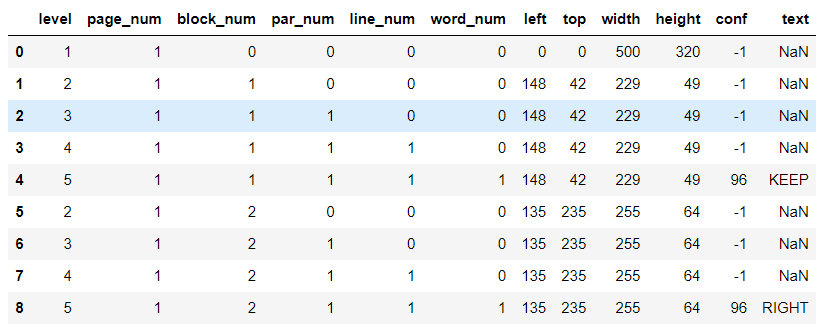Optical Character Recognition (OCR) is a technology used for extracting text data from images (both handwritten and typed). It is widely used for different kind of applications for extracting and using data for different purpose. There are different techniques used for processing of images and extract data from images using basic image processing, Machine learning and Deep learning techniques.

Tesseract is a open-source OCR engine owened by Google for performing OCR operations on different kind of images. It is written in C and C++ but can be used by other languages using wrappers and AddOns. We can use tesseract in python using `pytesseract` module which can be installed from PiP. So, for getting started, first we need to install tesseract binary on our system which can be downloaded from this url.

https://digi.bib.uni-mannheim.de/tesseract/

After installation, add its path to system environment variables so you can access it anywhere without specifiying path everytime.

``````# default path
C:\Program Files\Tesseract-OCR``````

Once you have installed and setup tesseract, you can verify it by typing `tesseract` in your command prompt and it will show its details. We can also use it using command line for getting text from images. Here is a simple demonstration to use it using cmd.

``````# base usage
tesseract IMAGE_PATH OPUTPUT_BASE

# example: it will process 'first.jpg' and store text in 'result.txt' file
tesseract first.png result``````

Now if we want to use it for processing multiple images and handle output, we can use pytesseract library which can be installed using command prompt.

``pip install pytesseract``

### Process Image

We can use both Pillow and OpenCV to read image files and input to pytesseract methods for processing images or we can directly provide image path to pytesseract but it has limitations. First, we will use direct method to read image file and input to pytesseract.

``````import pytesseract

# if you have not added tesseract exe to path
pytesseract.pytesseract.tesseract_cmd = "path_to_executable"

# extract text from image
resp = pytesseract.image_to_string(
"my_image.png"
)
print("Response:", resp)``````

You can also use other languages, to view list of languages available run following code.

``````laguages = pytesseract.get_languages(config='')
print(languages)``````
``['eng', 'osd']``

Now you can specify language you want to use in `image_to_string` method. Here is a list of supported input and output formats from pytesseract.

 JPEG PNG PBM PGM PPM TIFF BMP GIF WEBP

Supported output types: (default is string)

 string dict data.frame bytes

For more details on latest supported inputs and outputs, view details on github page below.

Now if we have some other image formats, we can read using pillow or opencv and then input to pytesseract method.

Using Pillow

``````from PIL import Image

my_image = Image.open('IMAGE_PATH')
# extract text from image
resp = pytesseract.image_to_string(
my_image
)
print("Response:", resp)``````

Using OpenCV

While using opencv, we need one more step as OpenCV read images in BGR format so we need to convert image to RGB format first.

``````import cv2

my_image  = cv2.cvtColor(my_image, cv2.COLOR_BGR2RGB) # convert from BGR to RGB

# extract text from image
resp = pytesseract.image_to_string(my_image)
print("Response:", resp)``````

### Batch Processing

We can also pass a list of images in a text file for batch processing.

``````resp = pytesseract.image_to_string('images.txt')
print(resp)``````

### Get BBOX

We can also get position for each detection in image. Later on we can use that data to extract those sub parts of image or draw on them for visualization. Here we will read using opencv and draw for each of the bbox position.

``````import cv2
my_image = cv2.cvtColor(main_image, cv2.COLOR_BGR2RGB)

print(bboxes)``````
``````K 148 229 197 278 0
E 212 229 256 278 0
E 270 229 315 277 0
P 328 229 377 277 0
R 135 34 184 83 0
I 196 34 209 83 0
G 221 21 272 85 0
H 282 35 332 84 0
T 345 35 390 84 0``````

Now we iterate over each detection and draw a rectangle using bbox coordinates. These values can be processed as follows.

``````h, w, _ = main_image.shape # we need main image shape
for row in resp.splitlines():
# split row to values
row = row.split(" ")
# draw rectangle
cv2.rectangle(
main_image,  # image
(int(row), h - int(row)),  # xmin, h-ymin
(int(row), h - int(row)), # xmax, h-ymax
(255, 0, 0), 2 # color and line size
)
# write text on each bbox
cv2.putText(main_image, row, (int(row), h - int(row) - 5), cv2.FONT_HERSHEY_DUPLEX, 1, (0, 255, 0), 2)
``````### Get Blocks

We can also get blocks detected by tesseract using `image_to_data` method. It returns more detailed data like bboxes, line numbers, confidence, page numbers and block so that we can perform post processing from tesseract. We can also get output as dictionary or pandas DataFrame. We will use dataframe so that we can process output easily.

``````# Get output as dataframe
image_data = pytesseract.image_to_data(my_image, output_type=pytesseract.Output.DATAFRAME)``````To get blocks, we can iterate over data and use only rows which are marked as blocks.

``````# iterate over rows where there is a word
for i, row in image_data[image_data.word_num==1].iterrows():
xmax = row.left+ row.width # left + width (xmax)
ymax =row.top + row.height # top + height (ymax)
cv2.rectangle(
main_image, (row.left, row.top),
(xmax, ymax), (255, 0, 0), 2
)
# write detected text of block
cv2.putText(main_image, row, (row.left, row.top - 5), cv2.FONT_HERSHEY_DUPLEX, 1, (0, 255, 0), 1)

cv2.imwrite("main_image.jpg", main_image)``````

### Searchable PDF

We can also store output as a pdf where we can search strings detected by pytesseract.

``````pdf = pytesseract.image_to_pdf_or_hocr(my_image, extension='pdf') # to pdf
with open('my_image.pdf', 'w+b') as f: # write as a pdf
f.write(pdf)``````

Now you can open and search using `ctrl+f` to find any string from pdf.

### Timeout

We can also specify a timeout which will cause pytesseract method to break after specified timeout.

``````try:
resp = pytesseract.image_to_string('test.jpg', timeout=1)) # Timeout after 1 second
except RuntimeError as timeout_error:
# Tesseract processing is terminated
print("Timeout:", str(timeout_error)``````

So, this way we can handle timeout while processing large dataset and different kind of images.

For more details on tesseract and pytesseract view following urls.